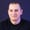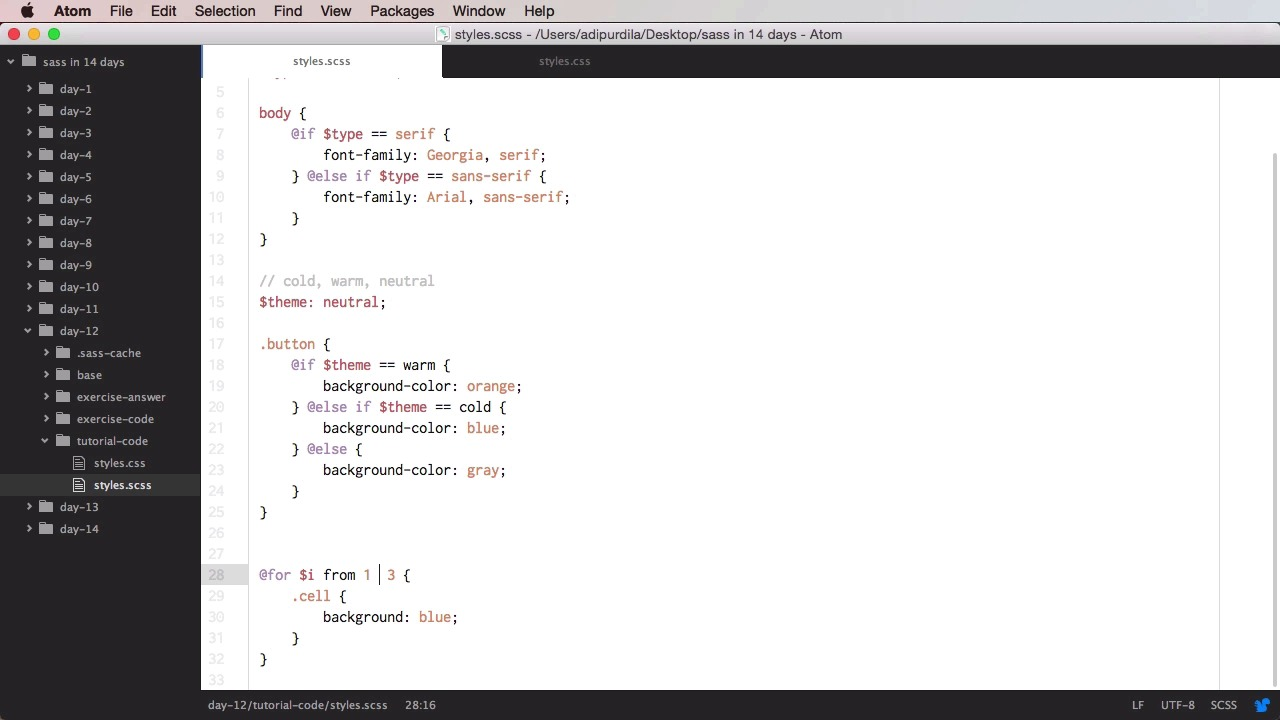Lessons: 20Length: 1.9 hours• Overview
• Transcript

# 4.5 Day 5: @if and @for Directives

Welcome to day #12, where you’ll learn about the @if and @for directives. @if is used for conditional statements, while @for will repeatedly output styles.

## 5.Conclusion1 lesson, 00:54

### 4.5 Day 5: @if and @for Directives

Welcome to day 12, where you'll learn about the if and for directives. The if is mainly used for conditional statements, while the for is used for repeatedly outputting certain styles. Let's begin. The if directive in SASS works just like a regular if statement in any language, JavaScript for example. If a condition is met then something happens. In our case the if directive takes an expression, evaluates it and if the expression returns anything other than false or null, it uses the styles under it. So, let's see an example here. Let's define, first of all a variable here. Type serif and let's say for example, that this can either be serif or sans-serif and then we have a body, and also something like this, if \$type, and here we're using the equality operator, == serif, then we're going to say something like, font-family and Georgia serif. So once we compile this, we can see our body has the font family Georgia serif but if I changed this to sans-serif or some other value then we don't get anything. We can also use the else director. So here we can say else if type equals sans serif then we are going to use the font family Ariel, sans serif. So now we have this but if we have some other value, we won't get anything because neither of these commissions are met. Now you can use as many else ifs as you want basically. So let's see another example. So let's say we have another theme here that can choose between let's say cold, warm, and neutral and we have a button, so if our theme is warm, then we're gonna set the background color to orange. Else if our theme is cold then the background color will be set to blue. Else, and this is without the if. We'll set our background color to gray. So what does this do? Well currently we have neutral selected, so it's not the first condition or the second condition. So if these two aren't met, we go to else and the if statement is going to use this style. So now we have background color gray. We can also add here an else if, else if theme is neutral, then use gray and then we can have another else and we just set a random color or maybe transparent but yeah that's it for the if directive. Now let's see about the for directive and the for directive will be repeatedly output a set of styles. So, for example, we're going to do four and then we're going to follow it with a variable name. In our case this is going to be a counter. So it's going to count the steps. We're just gonna say i from one to three for example. Let's do, for example, cell background blue and let's see what this compiles to. So we have cell, cell, so it compiles this two times. Now I have three here so why doesn't it compile three times? Well that's because the version with two here will not include the last element here so it will not include three. If I want to include that, I would do through. So we have two forms for this. We have from one to three which we'll do one and two and we have from one through three, which we'll do one, two and three, right? So let's comment this and let's see the result. Now we have cell printed three times. Now, you can also use variables here and you can do that without interpolation so for example let's define a comment count of ten and let's also do a for i from one to or let's use the other version through comment count and let's do this comment. Dash, and now I'm going to use the i variable to know which iteration I'm using. So I do have to use interpolation here, so I'm gonna use i. I'm gonna do before content, and also, again, with the interpolation i. So what this will do is it will compile into comment one, two, three, all the way to ten and it's gonna set its content to one all the way to ten. If I want, I can also go in to reverse. So for example from ten to one, for that I can do something like from comment-count through 1. So I start with a bigger value first and that will go. Comment ten, nine, eight, seven, all the way down to one. Now let's see another example. Let's say that I want to represent one of those signal icons where you have a short bar then right next to it you have a bigger bar and a bigger one and a bigger one, so you have a height that increases from bar to bar. Well that's actually quite easy to do let's say for example let's define a base style here bar, we set a width to it display inline block we set a background. Margin right to separate them and float to the left, so now what we can do is for i or j, it doesn't really matter how you name this variable from 1 through 4, we're gonna do something like bar j. We're gonna extend the bar, so as you can see you can combine these directives here and we'll set a height of let's say 1em times j. So that will compile to, bar-1 has a height of 1em, 2 2ems, 3 3ems, and 4 4ems. So this is another example of how we can use the four directive. Now, for the exercise, you have something that's a little bit more complex this time. You have an .sccs file and your assignment is to display a color palette that can have two themes, light and dark and each of the two themes has four colors represented by their hex codes. Now, the palette should be represented by four elements inside the pallet container and you're gonna find the code for that here and they should be absolutely positioned and displayed in a single line at 1em distance from one another. Also, you should use the least amount of SASS code possible. Good luck and that's it for these two directives. Now the four isn't the only directive that can be used to repeatedly output certain styles. You can also use the each and while directives and you'll learn about those in day 13.

Back to the top